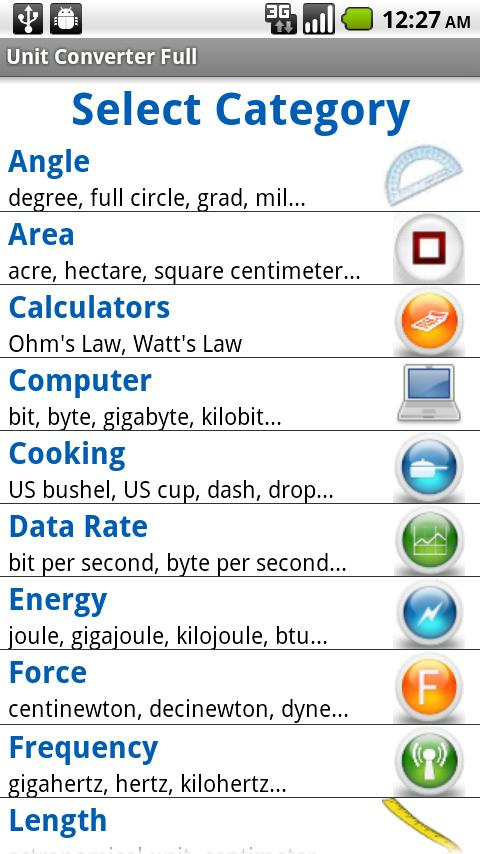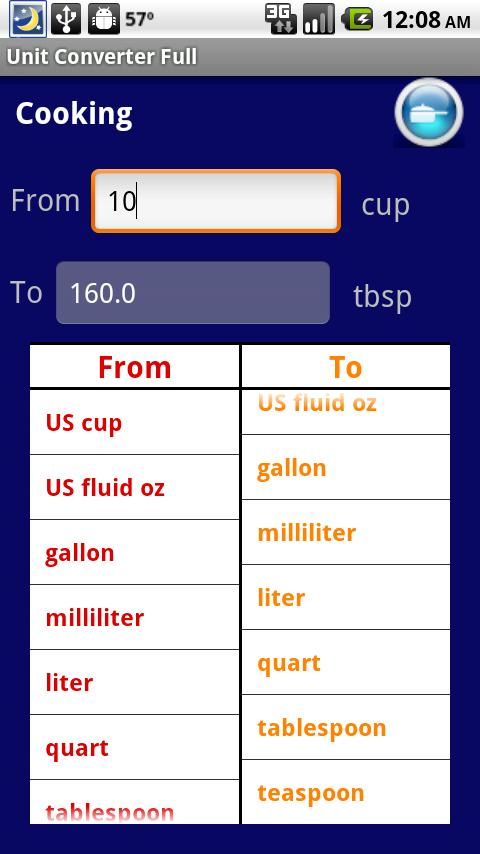# Unit Converter Full

## App Specification

• App Name: Unit Converter Full
• Rating: 4.25
• Apk: com.app.poz.unitconvert
• Cost: US\$0.99
• Market: market://search?q=pname:com.app.poz.unitconvert
•This simple unit converter tool allows you to easily convert among common everyday units as well as units you wouldnt think to convert.

Convert units such as length, weight, volume, and cooking to engineering units such as power, force, pressure, frequency, and energy. You can choose from many other different categories for converting units. Please see my website for the full list of units and categories implemented in this unit converter. Even has Ohms and Watts Law equations built in.

More categories and units to convert in future updates for this unit converter. This may not be the fanciest converter but is definitely one of the easiest one to use.

Feel free to email me suggestions for new categories and units to add to this unit converter tool.

** Please note the latest version now includes a fix to handle phones with smaller screen resolutions (320×480) such as the HTC Legend **

The following categories for converting units are available for this version:
- Angle
- Area
- Calculators (Ohms Law, Watts Law)
- Computer
- Cooking
- Data Rate
- Energy
- Force
- Frequency
- Length
- Power
- Pressure
- Speed
- Temperature
- Time
- Volume
- Weight

Tags: Unit Converter, unit converter, conversion tool, unit, converter, English to Metric converter, unit convert, unit converter, conversion tool, length to meters unit converter, units,unit converter, english unit converter, unit converter, conversions, units, unit, unit converter, unit converter, unit converter, unit converter full version, unit converter, unit converter, unit converter, unit converter, unit converter, unit converter, unit converter, unit converter, unit converter, unit converter, unit converter, unit converter, unit converter, unit converter, unit converter, conversion tool, conversion tool, conversion tool, conversion tool, unit converter, unit converter, unit converter, unit converter, units, units, converter, converter, converter, unit converter, unit converter, unit converter, unit converter, unit converter, unit converter, unit converter, unit converter, unit converter, unit converter, units, units, english, metric, unit converterPrevious Post: Math Calculators
Next Post: Vox Spanish Thesaurus TR